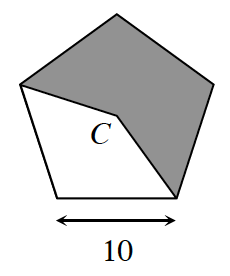### Home > CCG > Chapter 8 > Lesson 8.3.1 > Problem8-93

8-93.Find the area of the shaded region for the regular pentagon at right if the length of each side of the pentagon is $10$ units. Assume that point $C$ is the center of the pentagon.

Try dividing the pentagon into congruent triangles.
What trig ratio can you use to find the height of one of these triangles?
Use this height to find the area of one triangle, this is a good starting point to find the area of the shaded region.